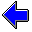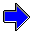Java Security Settings:
This web page employs Java, which requires specific security settings for correct operation.
If the applets on this page do not run correctly, consult the Virtual Chemistry Experiments FAQ
or the Physlet Physics web site for establishing the correct security settings.

# Spectrophotometry

## Beer's Law

In the previous two experiments, you observed that the absorbance varies linearly with both the cell path length and the analyte concentration. These two relationships can be combined to yield a general equation called Beer's Law.

A = ε l c

The quantity ε is the molar absorptivity; in older literature it is sometimes called the extinction coefficient. The molar absorptivity varies with the wavelength of light used in the measurement. The absorption spectrum is sometimes displayed in the form ε vs λ rather than A vs λ.

Conceptually, the transmittance is an easier quantity to understand than the absorbance. If T = 30%, then 30% of the photons passing through the sample reach the detector and the other 70% are absorbed by the analyte. The absorbance is a slightly less intuitive quantity. If A = 0, then no photons are absorbed. If A = 1.00, then 90% of the photons are absorbed; only 10% reach the detector. If A = 2.00, then 99% of the photons are absorbed; only 1% reach the detector. It is the absorbance, however, that displays a simple dependence on the concentration and cell path length (Beer's Law), and thus most chemists choose to report data in terms of absorbance rather than transmittance.

### Experiment

Objective:

• Determine the molar absorptivity for the analyte.

Approach:

• Measure the intensity of transmitted light for solutions with various concentrations of analyte.
• For each solution, calculate the absorbance of the solution.
• Construct a plot of A vs c.
• Plot the line-of-best-fit through the experimental points. The slope of this line is the molar absorptivity. The line should pass through the origin; that is, the intercept of this line should be zero.

The cell path length is 2.00 cm.

Run each simulation sufficiently long to detect at least 1000 photons. (Not all photons are shown on the screen.) Because the intensity for the blank is used to calculate all absorbances, it is especially important that the intensity for the blank be known accurately. If possible, wait until at least 4000 photons are detected for the blank.

The graph is constructed using concentrations in units of μmole L-1. The final molar absorptivity should have units of L mole-1 cm-1. You will need to perform the necessary unit conversion.

 Concentration: blank 13.7 μmole L-1 27.4 μmole L-1 41.1 μmole L-1 54.8 μmole L-1 Number of Photons Detected: Elapsed Time: sec Intensity of Detected Light: photons/sec LightSource Cell Detector c = μmole L-1     A =     Plotted Points:                                                                                                                                                                                                                                                                                                               slope =           intercept =Effect of Concentration                     Unknown SolutionSpectrophotometry Home PageVirtual Chemistry Home Page

BeersLaw.html version 2.1